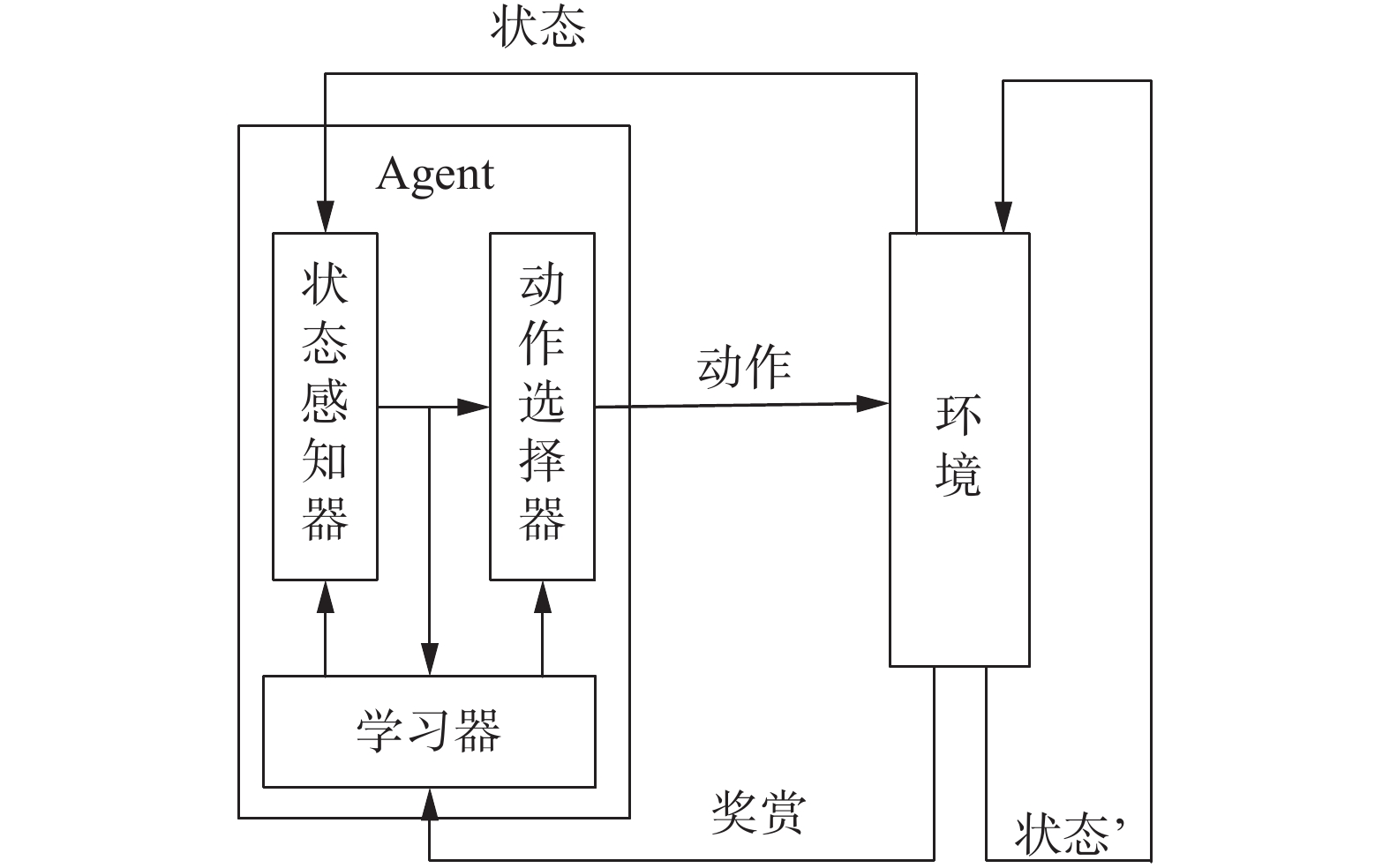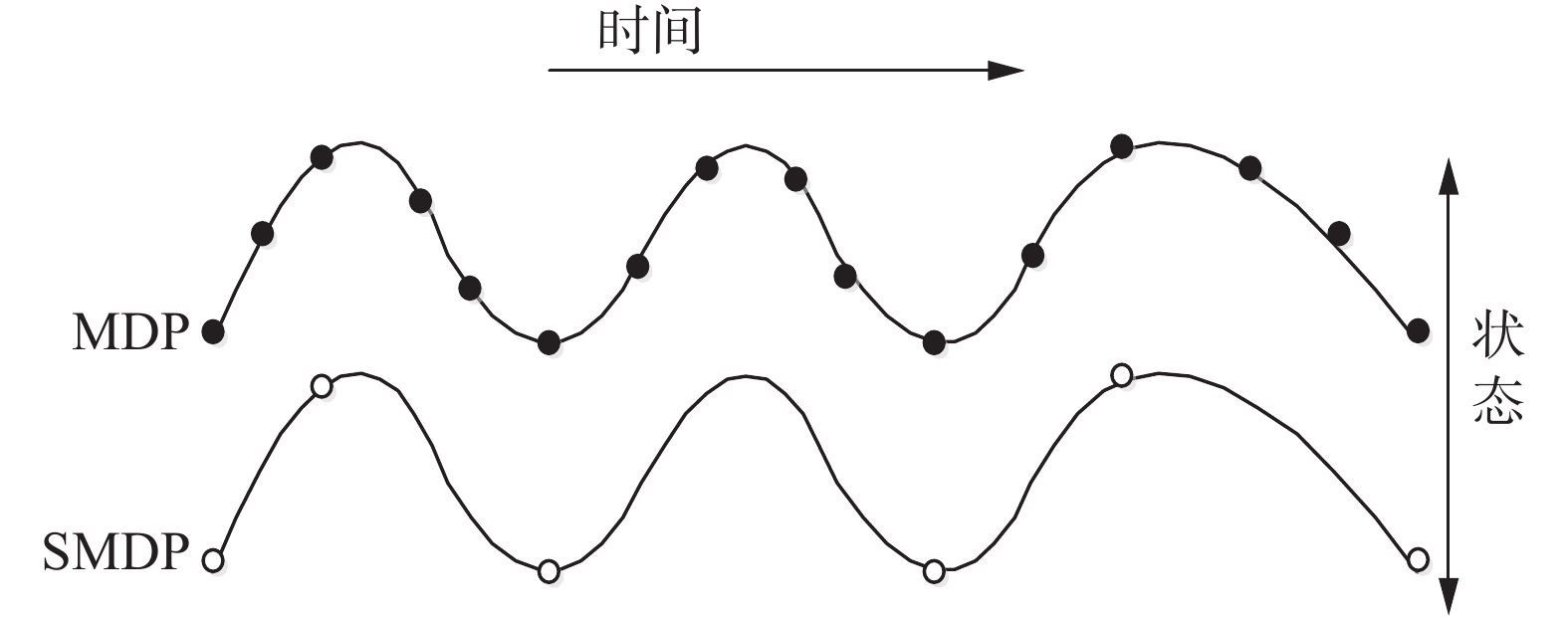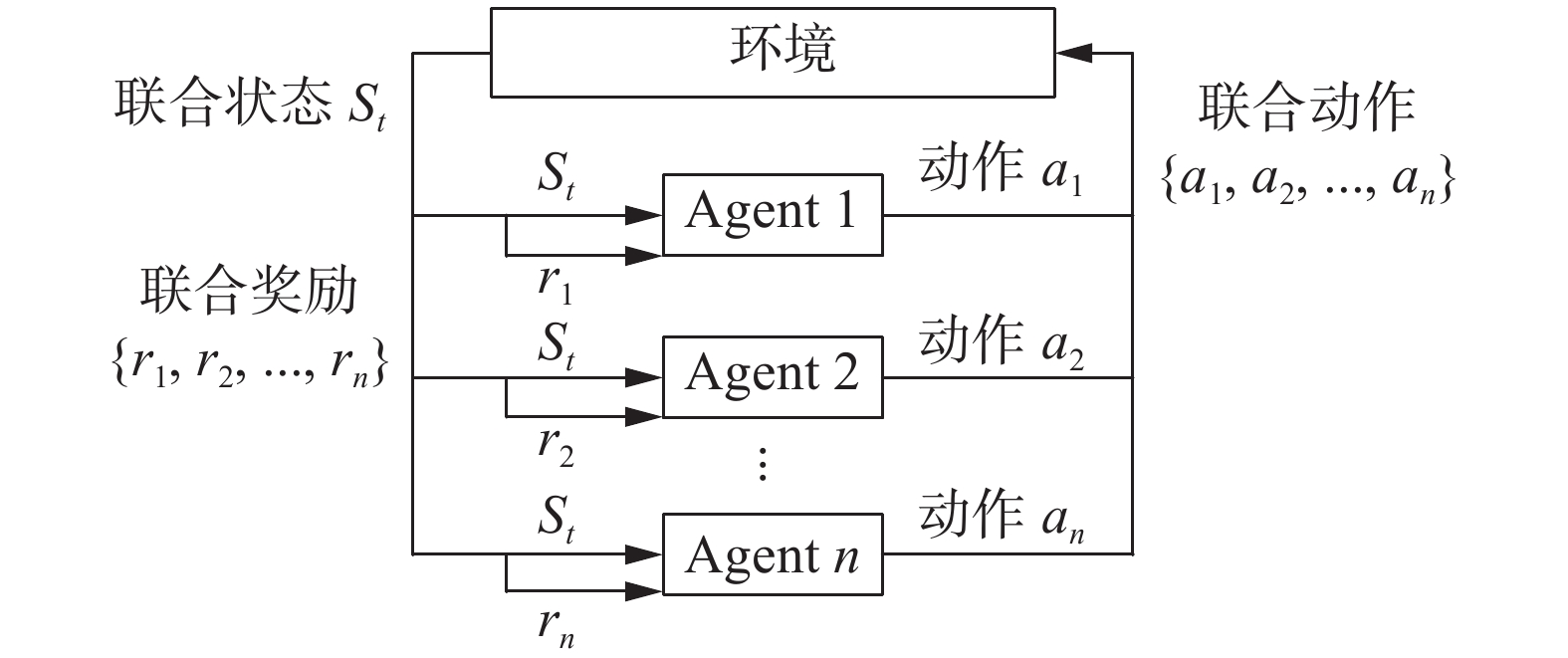﻿ 多智能体分层强化学习综述
«上一篇文章快速检索 高级检索

 智能系统学报2020, Vol. 15Issue (4): 646-655  DOI: 10.11992/tis.2019090270

### 引用本文YIN Changsheng, YANG Ruopeng, ZHU Wei, et al. A survey on multi-agent hierarchical reinforcement learning[J]. CAAI Transactions on Intelligent Systems, 2020, 15(4): 646-655. DOI: 10.11992/tis.201909027.### 文章历史

A survey on multi-agent hierarchical reinforcement learning
YIN Changsheng, YANG Ruopeng , ZHU Wei , ZOU Xiaofei , LI Feng
School of Information and Communication, National University of Defense Technology, Wuhan 430010, China
Abstract: As an important research area in the field of machine learning and artificial intelligence, multi-agent hierarchical reinforcement learning (MAHRL) integrates the advantages of the collaboration of multi-agent system (MAS) and the decision making of reinforcement learning (RL) in a general-purpose form, and decomposes the RL problem into sub-problems and solves each of them to overcome the so-called curse of dimensionality. So MAHRL offers a potential way to solve large-scale and complex decision problem. In this paper, we systematically describe three key technologies of MAHRL: reinforcement learning (RL), Semi Markov Decision Process (SMDP), multi-agent reinforcement learning (MARL). We then systematically describe four main categories of the MAHRL method from the angle of hierarchical learning, which includes Option, HAM, MAXQ and End-to-End. Finally, we end up with summarizing the application status of MAHRL in robot control, game decision making and mission planning.
Key words: artificial intelligence    machine learning    reinforcement learning    multi-agent    summary    reinforcement learning    hierarchical reinforcement learning    application status

1 预备知识 1.1 强化学习Download: 图 1 强化学习的框架结构 Fig. 1 Framework structure of reinforcement learning

Agent的动作会对环境产生影响，其环境状态也会发生变化，此时Agent会收到来自环境的反馈信号，分别用正负反馈表示这个动作对学习目标是否有益。Agent则通过不断地试错和反馈来不断优化动作选择策略，最终学习到一个有目标导向的策略。

1)基于值函数的强化学习。其核心思想是采用函数近似的方法将强化学习模型中的状态值函数、状态动作值函数或策略函数用一个显性的函数来表示，常用的近似函数有线性函数近似、决策树近似、核函数近似和神经网络等。其中深度神经网络是近年来在强化学习值函数近似方面应用最为广泛和成功的函数。其中,最为典型的应用开始于2013年DeepMind团队在NIPS上提出的DQN(deep Q-networks)算法，其基于值函数的泛化逼近方法，有效解决了强化学习的“维数灾难问题”，但在Q函数逼近过程中存在不稳定的现象。为解决部分可观察的马尔可夫决策问题，Hausknecht等提出了基于循环神经网络与强化学习相结合的DRQN算法，在实验环境中取得了远优于DQN算法的效果。典型的值函数近似方法还有DDQN、Sarsa、Q-learning等时序差分的强化学习算法，它们虽然在某些实际问题中取得了不错的效果，但其难以求解动作空间比较大和随机性策略问题，以及无法对连续动作空间问题进行建模等。

2)基于策略搜索的强化学习。其核心思想是将策略参数化，通过不断修正策略的参数求解最优策略。Silver等结合Actor-Critic框架和Q-learning算法提出了确定性策略梯度算法(deterministic policy gradient algorithms, DPG)，其将策略定义为一个确定性的策略函数，这样在训练模型时，就不需要考虑动作空间的大小或是否连续，能够提升连续动作空间问题求解中对于梯度的估计效率和准确性。针对经验回放的深度强化学习方法存在对内存和计算能力要求较高的问题，Mnih等提出了异步梯度下降的深度Actor-Critic框架，用于并行执行多个智能体用于神经网络控制器的优化，并与单步Q-learning、Sarsa、多步Q-learning和Actor-Critic等强化学习算法结合实现了对深度神经网络的训练，并取得了更好的效果。除此之外还有可信赖域策略搜索算法TRPO 、价值梯度SVG、引导策略搜索算法GPS、近端策略优化算法PPO和广义优势估计算法GAE随机等几种典型的基于策略梯度的方法。

3)基于环境建模的强化学习。其核心思想是通过建立环境模型来产生模拟经验样本。对于某些智能体与环境的交互成本较高的情况，而基于环境建模的强化学习通过建立环境模型来模拟经验样本，可以减少采样次数，或者无需在真实环境中进行采样。Sutton提出的Dyna框架是一种典型的基于环境建模的强化学习。其基本思想是利用与真实环境产生的经验样本来进行环境模型的学习，而值函数或者策略函数的学习与更新则基于真实样本和环境模型产生的虚拟样本。虽然Dyna框架能基于真实样本来进行环境模型学习，并取得了不错的效果，但其环境模型的建立和真实经验样本和虚拟样本的权衡仍然是制约该方法发展的关键难点。

1.2 半马尔可夫决策过程Download: 图 2 MDP与SMDP Fig. 2 MDP & SMDP

 $\begin{array}{c} {V^\pi }\left( s \right) = \bar R\left( {s,\pi \left( s \right)} \right) + \\ \displaystyle\sum \nolimits_{s',N} P(s',N|s,\pi \left( s \right)){\gamma ^N}{V^\pi }\left( {s'} \right) \end{array}$ (1)

1.3 多智能体强化学习Download: 图 3 多智能体强化学习示意图 Fig. 3 Multi-agent reinforcement learning diagram

1)多智能体系统研究。当前多智能体系统研究主要从体系结构、Agent信息交互方式和Agent冲突消解机制3个方面展开。①体系结构研究：主要围绕集中式和分散式2种展开研究。其中分散式又可以区分为层面式(distributed)与分层式(hierarchical)。除了基本的分层方式，多智能体体系结构研究还包括智能体协同和任务分配机制等。②智能体之间信息交互方式研究：目前主要有Agent直接通信、信道广播方式、信息黑板模式等方法。③智能体间冲突消解研究：多智能体系统中冲突矛盾主要包括空间冲突、信息冲突和任务冲突等。当前消解矛盾方法主要包括集中控制模块法和主从控制法2种。集中控制模块法通过构建模块来集中规划所有Agent的行动策略，但是会带来通信阻塞问题。主从控制法是指通过一个中心智能体来统一进行任务分配和行为规划来解决智能体之间的矛盾问题，但由于实时性和灾难空间等问题，其适应性和鲁棒性有待提升。

2)多智能体强化学习策略研究。目前MARL的学习策略主要可以分为3类：基于共享、基于对策和基于最佳响应的多智能体强化学习。①基于共享的MARL：其主要思想是研究动作选择前Agent之间的相互交互、信息共享以及值函数更新方法，基于分布式强化学习提高学习速度，典型算法有状态共享、经验共享、策略共享和建议共享等。②基于对策的MARL：其主要思想是以对策论为基础，综合考虑所有Agent的值函数，寻求某种对策下的平衡来选择动作，代表算法有Minimax-Q、Nash-Q、WoLF、CE-Q等。③基于最佳响应的MARL：其主要思想是在其他Agent无论采取何种策略情况下寻求最优策略，算法主要依赖于收敛准则和无憾准则，典型算法有PHC、IGA、GIGA、GIGA-WoLF等。

2 多智能体分层强化学习方法

MAHRL是MARL和HRL相结合的结果。二者结合有两种思路：一是基于分层来解决MARL问题，二是采用多智能体解决HRL问题，所以现有MAHRL可根据采用的HRL方法或者MARL方法等不同角度进行分类。由于目前的研究多集中于前者，本文从分层强化学习的角度对多智能体分层强化学习方法进行探讨，即基于选项(option)、基于分层抽象机(hierarchical of abstract machines，HAM)、基于值函数分解(MaxQ value function decomposition)和基于端到端的(end to end)多智能体分层强化学习。

2.1 基于选项的多智能体分层强化学习

Markov-Option $<\varphi ,\pi ,\beta >$ 三元组分别代表Option的入口状态集、内部策略和终止条件。Option开始执行的前提条件是当前状态属于入口状态集，即 $s\subseteq \varphi$ ，其中入口状态集 $\varphi \subseteq S$ 。内部策略 $\pi$ 一般表示为 $\pi :\varphi \times {A}_{\varphi }\to \left[\mathrm{0,1}\right]$ ，其中 ${A}_{\varphi }$ 为在入口状态集 $\varphi$ 上能够执行的基本动作集。终止条件 $\beta$ 为基于状态 ${s}'$ 终止的概率集合 $\beta \left({s}'\right)$ ，一般表示为 $\beta :S\to \left[\mathrm{0,1}\right]$ 。因此 $\; \beta \left({s}_{G}\right)=1$ 一般作为Option任务的子目标状态点 ${s}_{G}$ 的终止条件，同时 ${A}_{\varphi }$ 可以视为Markov-Option的一种特例。

Semi-Markov-Option $<\varphi ,\mu ,\beta >$ 三元组含义类似。同样其Option开始执行的前提条件 $s\subseteq \varphi$ ，其中入口状态集 $\varphi \subseteq S$ ，且其只能包含该Option可能探索到的所有状态。内部策略为 $\mu :\varphi \times {O}_{\varphi }\to \left[\mathrm{0,1}\right]$ ，其中 ${O}_{\varphi }$ 为在入口状态集 $\varphi$ 上能够执行的基本动作集。终止条件 $\beta$ 为基于状态 ${s}'$ 终止的概率集合 $\beta \left({s}'\right)$ ，而 $\beta \left({s}_{G}\right)=1$ 通常也是Option任务的子目标状态点 ${s}_{G}$ 的终止条件。

 $\begin{array}{c} \;\;\;\;\;\;\;\;\;\;\;R\left( {s,o} \right) = \\ E\left\{ {{r_t} + \gamma {r_{t + 1}} + \cdots + {\gamma ^{\tau - 1}}{r_{t + \tau - 1}}|\varepsilon (o,s,t)} \right\} \end{array}$ (2)

 $P\left( {s'|s,o} \right) = \sum\limits_{\tau = 1}^\infty {{\gamma ^\tau }} P(s',\tau )$ (3)

 $\begin{array}{l} {Q_{k + 1}}\left( {s,o} \right) = \left( {1 - {\alpha _k}} \right){Q_k}\left( {s,o} \right) + \\ \;\;\;\;{\alpha _k}\left[ {r + {\gamma ^\tau }{\rm{ma}}{{\rm{x}}_{o' \in {O_{s'}}}}{Q_k}(s',o')} \right] \end{array}$ (4)

Agent的每次学习执行都是以一个Option终止为前提，故造成其利用率不高，为此Precup基于Markov-Option提出了一种面向单时间步Q-Learning的Option方法，并证明了其收敛性。Tang等则针对StarCraft游戏问题，根据作战规则不同，作战要素和动作空间组合不同设计101种输入特征向量选项，实现了订单生产的强化学习。

2.2 基于分层抽象的多智能体分层强化学习

 $\begin{array}{l} {Q_{k + 1}}\left( {\left[ {{s_c},{m_c}} \right],{a_c}} \right) = \left( {1 - {\alpha _k}} \right){Q_k}\left( {\left[ {{s_c},{m_c}} \right],{a_c}} \right) + \\ \;\;\;\;\;\;{\alpha _k}[{r_t} + \gamma {r_{t + 1}} + \cdots + {\gamma ^{\tau - 1}}{r_{t + \tau - 1}} + \\ \;\;\;\;\;\;\;\;\;\;\;\;\;\;{\gamma ^\tau }{\rm{max}}{Q_k}(\left[ {{s_{c'}},{m_{c'}}} \right],a')] \end{array}$ (5)

2.3 基于值函数分解的多智能体分层强化学习

 $\begin{array}{l} \;\;\;\;{V^\pi }\left( {i,s} \right) = {V^\pi }\left( {a,s} \right) + \\ \displaystyle\sum\nolimits_{s',\tau } {P_i^\pi } (s',\tau |s,a){\gamma ^\tau }{V^\pi }(i,s') \end{array}$ (6)

 $\begin{array}{l} \;\;\;\;{Q^\pi }\left( {i,s,a} \right) = {V^\pi }\left( {a,s} \right) + \\ \displaystyle\sum\nolimits_{s',\tau } {P_i^\pi } (s',\tau |s,a){\gamma ^\tau }{\rm{max}}{Q^\pi }(i,s',\pi \left( {s'} \right)) \end{array}$ (7)

 ${C^\pi }\left( {i,s,a} \right) = \sum\nolimits_{s',\tau } {P_i^\pi } (s',\tau |s,a){\gamma ^\tau }{\rm{max}}{Q^\pi }(i,s',\pi \left( {s'} \right))$

 ${Q}^{\pi }\left(i,s,a\right)={V}^{\pi }\left(a,s\right)+{C}^{\pi }\left(i,s,a\right)$ (8)

 $\begin{array}{l} {V^\pi }\left( {0,s} \right) = {V^\pi }\left( {{a_n},s} \right) + {C^\pi }\left( {{a_{n - 1}},s,{a_n}} \right) + \cdots + \\ \;\;\;\;\;\;\;\;\;\;\;\;\;{C^\pi }\left( {{a_1},s,{a_2}} \right) + {C^\pi }\left( {0,s,{a_1}} \right) \end{array}$ (9)

2.4 基于端到端的多智能体分层强化学习

3 多智能体分层强化学习的应用 3.1 多智能体分层强化学习在机器人控制领域的应用

3.2 多智能体分层强化学习在博弈决策领域的应用

3.3 多智能体分层强化学习在任务规划领域的应用

4 结束语

  LECUN Y, BENGIO Y, HINTON G. Deep learning[J]. Nature, 2015, 521: 436-444. DOI:10.1038/nature14539 (0)  SILVER D, HUBERT T, SCHRITTWIESER J, et al. A general reinforcement learning algorithm that masters chess, shogi, and Go through self-play[J]. Science, 2018, 362: 1140-1144. DOI:10.1126/science.aar6404 (0)  JADERBERG M, CZARNECKI M M, DUNNING L, et al. Human-level performance in 3D multiplayer games with population-based reinforcement learning[J]. Science, 2019, 364(6443): 859-865. DOI:10.1126/science.aau6249 (0)  LIU Siqi, LEVER G, MEREL J, HEESS N, et al. Emergent coordination through completion[EB/OL]. [2019-2-21]. https://arxiv.org/abs/1902.07151. (0)  WU Bin, FU Qiang, LIANG Jing, et al. Hierarchical macro strategy model for MOBA game AI[EB/OL]. [2018-12-19]. https://arxiv.org/abs/1812.07887v1. (0)  MNIH V, KAVUKCUOGLU K, SILVER D, et al. Playing atari with deep reinforcement learning[EB/OL]. [2013-12-19]. https://arxiv.org/abs/1312.5602. (0)  WOOLDRIDGE M. An introduction to multi-agent systems[J]. Wiley & Sons, 2011, 4(2): 125-128. (0)  GIL P, NUNES L. Hierarchical reinforcement learning using path clustering[C]//Proceedings of 8th Iberian Conference on Information Systems and Technologies. Lisaboa, Portugal, 2013: 1−6. (0)  XUE B, GLEN B. DeepLoco: dynamic locomotion skills using hierarchical deep reinforcement learning[J]. ACM transactions on graphics, 2017, 36(4): 1-13. (0)  SUTTON R S, BARTO A G. Reinforcement learning: an introduction[M]. Cambridge: MIT Press, 1998. (0)  SILVER D, SCHRITTEIESER J, SIMONYAN K, et al. Mastering the game of go without human knowledge[J]. Nature, 2017, 550(7676): 354-391. DOI:10.1038/nature24270 (0)  刘全, 翟建伟, 章宗长, 等. 深度强化学习综述[J]. 计算机学报, 2018, 41(1): 1-27. LIU Quan, ZHAI Jianwei, ZHANG Zongchang, et al. A survey on deep reinforcement learning[J]. Chinese journal of computers, 2018, 41(1): 1-27. DOI:10.11897/SP.J.1016.2019.00001 (0)  HAUSKNECHT M, STONE P. Deep recurrent q-learning for patially observable mdps[EB/OL]. [2017-11-16]. https://arxiv.org/abs/1507.06527. (0)  HASSELT H V, GUEZ A, SILVER D. Deep reinforcement learning with double Q learning[EB/OL]. [2015-12-8]. https://arxiv.org/abs/1509.06461v1. (0)  RUMMERY G A, NIRNJAN M. On-line q-learning using connectionist systems[EB/OL]. [2018-2-2]. https://www.researchgate.net/publication/250611_On-Line_Q-Learning_Using_Connectionist_Systems. (0)  WATKINS C, DAYAN P. Q-learning[J]. Machine learning, 1992, 8(34): 279-292. (0)  SILVER D, LEVER G, HEESS N, et al. Deterministic policy gradient algorithms [C]//International Conference on Machine Learning 2014. Beijing, China, 2014: 387−395. (0)  MNIH V, BADIA A P, MIRZA M, et al. Asynchronous methods for deep reinforcement learning [EB/OL]. [2016-6-16]. https://arxiv.org/abs/1602.01783. (0)  SCHULMAN J, LEVINE S, ABBEEL P, et al. Trust region policy optimization [EB/OL]. [2015-2-19]. https://arxiv.org/abs/1502.05477. (0)  HEESS N, WAYNE G, SILVER D, et al. Learning continuous control policies by stochastic value gradients[EB/OL]. [2015-10-30]. https://arxiv.org/abs/1510.09142. (0)  LEVINE S, KOLTUM V. Guided policy search[EB/OL]. [2016-10-3]. https://arxiv.org/abs/1610.00529. (0)  SCHULMAN J, WOLSKI F, DHARIWAL P, et al. Proximal policy optimization algorithms[EB/OL]. [2018-9-18]. https://arxiv.org/abs/1707.06347. (0)  SCHULMAN J, MORITZ P, LEVINE S, et al. High dimensional continuous control using generalized advantage estimation [EB/OL]. [2011-11-16]. https://arxiv.org/abs/1506.024398. (0)  SUTTON R S. Dyna, an integrated architecture for learning, planning and reacting[J]. ACM SIGART bulletin, 1991, 2(4): 160-163. DOI:10.1145/122344.122377 (0)  DING Shifei, ZHAO Xingyu, XU Xinzheng, et al. An effective asynchronous framework for small scale reinforcement learning problems[J]. Applied intelligence, 2019, 49(12): 4303-4318. DOI:10.1007/s10489-019-01501-9 (0)  ZHAO Xingyu, DING Shifei, AN Yuexuan, et al. Applications of asynchronous deep reinforcement learning based on dynamic updating weights[J]. Applied intelligence, 2019, 49(2): 581-591. DOI:10.1007/s10489-018-1296-x (0)  ZHAO Xingyu, DING Shifei, AN Yuexuan, et al. Asynchronous reinforcement learning algorithms for solving discrete space path planning problems[J]. Applied intelligence, 2018, 48(12): 4889-4904. DOI:10.1007/s10489-018-1241-z (0)  SUTTON R S, PRECUP D, SINGH S R. Between MDPs and Semi-MDPs: a framework for temporal abstraction in reinforcement learning[J]. Artificial intelligence, 1999, 112(1-2): 181-211. DOI:10.1016/S0004-3702(99)00052-1 (0)  PRECUP D, SUTTON R S. Multi-time models for temporally abstract planning[C]// Proceedings of the 1997 Conference on Advances in Neural Information Processing Systems 10. Cambridge, United States, 1998: 1050−1056. (0)  PRECUP D. Temporal abstraction in reinforcement learning. [D]. Amherst: University of Massachusetts, USA, 2000. (0)  TANG Zhentao, ZHAO Dongbin, ZHU Yuanheng. Reinforcement learning for build-order production in StarCraft II [C]//8th International Conference on Information Science and Technology. Istanbul, Turkey. 2018. (0)  PARR R. Hierarchical control and learning for markov decision processes[D]. Berkeley: University of California, 1998. (0)  KULKARNI T D, NARASIMHAN K R, SAEEDI A, et al. Hierarchical deep reinforcement learning: integrating temporal abstraction and intrinsic motivation[EB/OL]. [2016-4-20]. https://arxiv.org/abs/1604.06057. (0)  DIETTERICH T G. Hierarchical reinforcement learning with the MAXQ value function decomposition[J]. Journal of artificial intelligence research, 2000, 13: 227-303. DOI:10.1613/jair.639 (0)  MENACHE I, MARMOR S, SHIMKIN N. Q-Cut: dynamic discovery of sub-goals in reinforcement learning[J]. Lecture notes in computer science 2430.2002: 295−306. (0)  DRUNNOND C. Accelerating reinforcement learning by composing solutions of automatically identified subtasks[J]. Journal of artificial intelligence research, 2002, 16: 59-104. DOI:10.1613/jair.904 (0)  HENGST B. Discovering hierarchy in reinforcement learning[D]. Sydney: University of New South Wales, Australia, 2003. (0)  UTHER W T B. Tree based hierarchical reinforcement learning[D]. Pittsburgh: Carnegie Mellon University, USA, 2002. (0)  PIERRE B, JEAN H. The option-critic architecture[C]//Proceedings of 31th AAAI Conference on Artifical Intelligence. San Francisco, USA, 2017: 1726−1734. (0)  VEZHNEVETS A S, OSINDERO S, SCHAUL T, et al. Feudal networks for hierarchical reinforcement learning[C]//Proceedings of 34th International Conference on Machine Learning. Sydney, Australia, 2017: 3540−3549. (0)  PONSEN M J V, SPRONCK P, AHA D W. Automatically acquiring domain knowledge for adaptive game AI using evolutionary learning[C]//Conference on Innovative Applications of Artificial Intelligence. Pittsburgh, Pennsylvania, 2005: 1535−1540. (0)  WEBER B G, ONTANON S. Using automated replay annotation for case-based planning in games[C]//18th International Conference on Case-based Reasoning. Alessandria, Italy, 2010: 15−24. (0)  WEBER B G, MAWHORTER P, MATEAS M, et al. Reactive planning idioms for multi-scale game AI[C]// Conference on Computational Intelligence and Games, Maastricht, The Netherlands, 2010: 115−122. (0)  SONG Y, LI Y, LI C. Initialization in reinforcement learning for mobile robots path planning[J]. Control theory & applications, 2012, 29(12): 1623-1628. (0)  LIU Chunyang, TAN Yingqing, LIU Changan, MA Yingwei. Application of multi-Agent reinforcement learning in robot soccer[J]. Acta electronica sinica, 2010, 38(8): 1958-1962. (0)  DUAN Yong, CUI Baoxia, XU Xinhe. Multi-agent reinforcement learning and its application role assignment of robot soccer[J]. Control theory & app1ications, 2009, 26(4): 371-376. (0)  SYNNAEVE G, BESSIERE P. A bayesian model for RTS units control applied to starcraft[J]. IEEE transactions on computational intelligence and AI in games, 2011, 3(1): 83-86. DOI:10.1109/TCIAIG.2011.2128770 (0)  SURDU J R, KITTKA K. Deep green: commander’s tool for COA’s concept[C]//Computing, Communications and Control Technologies 2008, Orlando, Florida, USA, 2008. (0)  ERNEST N, CARROLL D, SCHUMACHER C, et al. Genetic fuzzy based artificial intelligence for unmanned combat aerial vehicle control in simulated air combat missions[J]. Journal of denfense management, 2016, 6(1): 1-7. (0)  DERESZYNSKI E, HOSTETLER J, FERN A, et al. Learning probabilistic behavior models in real-time strategy games[C]//Proc of the 7th AAAI Conference on Artificial Intelligence and Interactive Digital Entertainment, Stanford, USA, 2011: 20−25. (0)  胡桐清, 陈亮. 军事智能辅助决策的理论与实践[J]. 军事系统工程, 1995(C1): 3-10. HU Tongqing, CHEN Liang. Theory and practice of military intelligence assistant decision[J]. Military operations research and systems engineering, 1995(C1): 3-10. (0)  朱丰, 胡晓峰. 基于深度学习的战场态势评估综述与研究展望[J]. 军事运筹与系统工程, 2016, 30(3): 22-27. ZHU Feng, HU Xiaofeng. Overview and research prospect of battlefield situation assessment based on deep learning[J]. Military operations research and systems engineering, 2016, 30(3): 22-27. DOI:10.3969/j.issn.1672-8211.2016.03.004 (0)  TIAN Yuandong, GONG Quchengg, SHANG Wenling, et al. ELF: an extensive, lightweight and flexible research platform for real-time strategy games [C]//31st Conference and Workshop on Neural Information Processing Systems, California, USA, 2017: 2656−2666. (0)  MEHTA M, ONTANOS S, AMUNDESEN T, et al. Authoring behaviors for games using learning from demonstration[C]//Proc of the 8th Intenational Conference on Case-based Reasoning, Berlin, Heidelberg, 2009: 12−20. (0)  JUSTESEN N, RISI S. Learning macromanagement in StarCraft from replays using deep learning[C]// IEEE’s 2017 Conference on Computational Intelligence in Games, New York, USA. 2017. (0)  WU Huikai, ZHANG Junge, HUANG Kaiqi. MSC: A dataset for macro-management in StarCraft II [DB/OL]. [2018-05-31]. http://cn.arxiv.org/pdf/1710.03131v1. (0)  BATO A G, MAHADEVAN S. Recent advances in hierarchical reinforcement learning[J]. Discrete event dynamic systems, 2013, 13(4): 341-379. (0)  TIMOTHY P L, JONATHAN J H, PRITZEL A, et al. Continous control with deep reinforcement learning[EB/OL]. [2015-11-18]. https://arxiv.org/abs/1509.02971. (0)  DIBIA V, DEMIRALP C. Data2Vis automatic generation of data visualizations using sequence to sequence recurrent neural networks [EB/OL]. [2018-11-2]. https://arxiv.org/abs/1804.03126. (0)  SUSHIL J L, LIU Siming. multi-objective evolution for 3D RTS micro [EB/OL]. [2018-3-8]. https://arxiv.org/abs/1803.02943. (0)  PENG Peng, WEN Ying, YANG Yaodong, et al. Multiagent bidirectionally-coordinated nets: emergence of human-level coordination in learning to play StarCraft combat games[EB/OL]. [2018-05-31]. http://cn.arxiv.org/pdf/1703.10069v4. (0)  SHAO Kun, ZHU Yuanheng, ZHAO Dongbin. StarCraft micromanagement with reinforcement learning and curriculum transfer learning[J]. IEEE transactions on emerging topics in computational intelligence, 2018(99): 1-12. (0)  李耀宇, 朱一凡, 杨峰. 基于逆向强化学习的舰载机甲板调度优化方案生成方法[J]. 国防科技大学学报, 2013, 35(4): 171-175. LI Yaoyu, ZHU Yifan, YANG Fan. Inverse reinforcement learning based optimal schedule generation approach for carrier aircraft on flight deck[J]. Journal of national university of defense technology, 2013, 35(4): 171-175. DOI:10.3969/j.issn.1001-2486.2013.04.030 (0)  陈希亮, 张永亮. 基于深度强化学习的陆军分队战术决策问题研究[J]. 军事运筹与系统工程, 2017, 31(3): 20-27. CHEN Xiliang, ZHANG Yongliang. Research on tactical decision of army units based on deep reinforcement learning[J]. Military operations research and systems engineering, 2017, 31(3): 20-27. DOI:10.3969/j.issn.1672-8211.2017.03.004 (0)  乔永杰, 王欣九, 孙亮. 陆军指挥所模型自主生成作战计划时间参数的方法[J]. 中国电子科学研究院学报, 2017, 12(3): 278-284. QIAO Yongjie, WANG Xinjiu, SUN Liang. A Method for Army command post to auto-Generate combat time scheduling[J]. Journal of china academy of electronics and information technology, 2017, 12(3): 278-284. DOI:10.3969/j.issn.1673-5692.2017.03.011 (0)  DING Shifei, DU Wei, ZHAO Xingyu, et al. A new asynchronous reinforcement learning algorithm based on improved parallel PSO[J]. Applied intelligence, 2019, 49(12): 4211-4222. DOI:10.1007/s10489-019-01487-4 (0)  ZHENG Yanbin, LI Bo, AN Deyu, et al. Multi-agent path planning algorithm based on hierarchical reinforcement learning and artificial potential field[J]. Journal of computer applications, 2015, 35(12): 3491-3496. (0)  王冲, 景宁, 李军, 等. 一种基于多Agent强化学习的多星协同任务规划算法[J]. 国防科技大学学报, 2011, 33(1): 53-58. WANG Chong, JING Ning, LI Jun, et al. An algorithm of cooperative multiple satellites mission planning based on multi-agent reinforcement learning[J]. Journal of national university of defense technology, 2011, 33(1): 53-58. DOI:10.3969/j.issn.1001-2486.2011.01.012 (0)